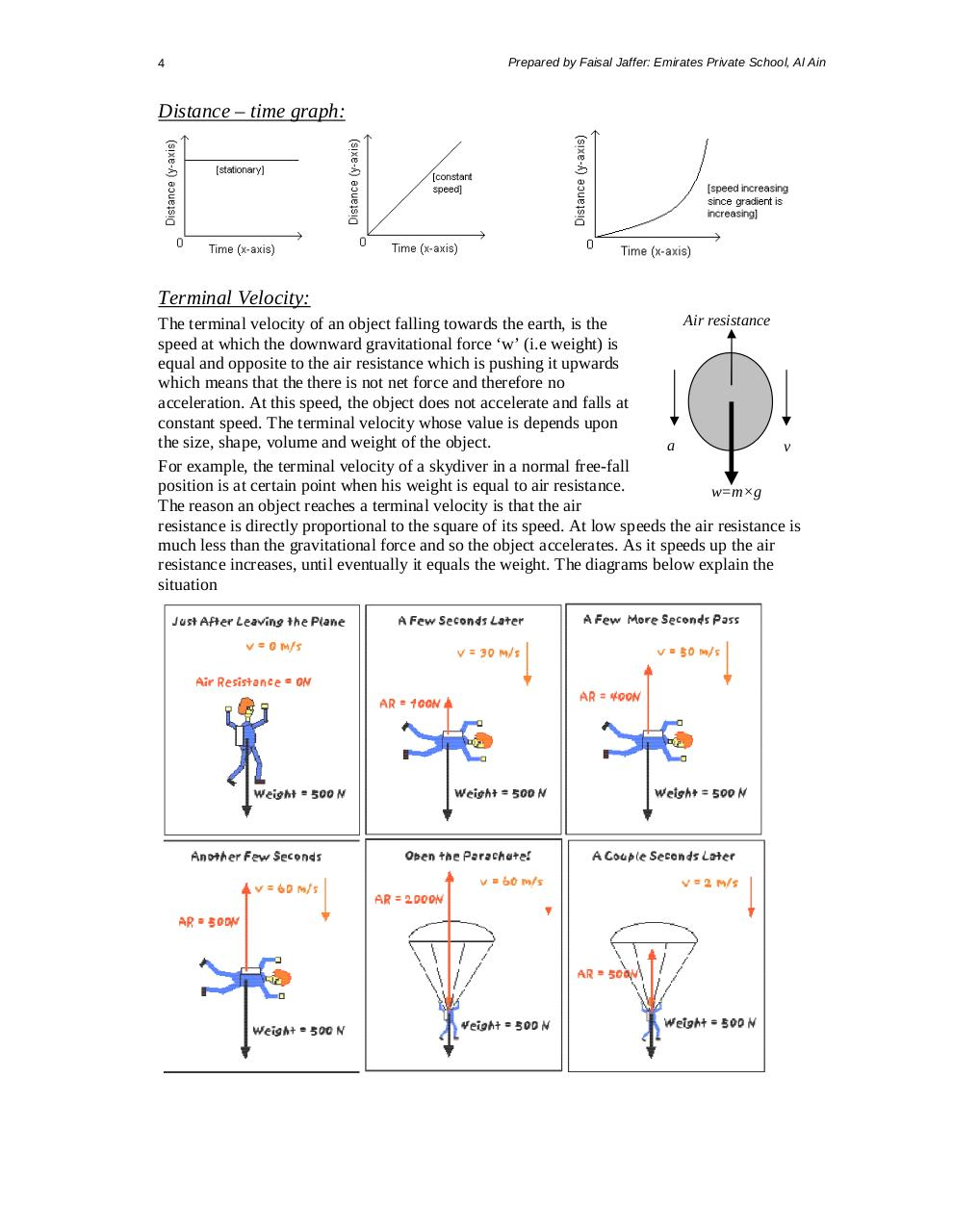# Summary of Chapter 2,3,4.pdfPage 1 2 3 45617

#### Text preview

4

Prepared by Faisal Jaffer: Emirates Private School, Al Ain

Distance – time graph:

Terminal Velocity:
Air resistance
The terminal velocity of an object falling towards the earth, is the
speed at which the downward gravitational force ‘w’ (i.e weight) is
equal and opposite to the air resistance which is pushing it upwards
which means that the there is not net force and therefore no
acceleration. At this speed, the object does not accelerate and falls at
constant speed. The terminal velocity whose value is depends upon
the size, shape, volume and weight of the object.
a
v
For example, the terminal velocity of a skydiver in a normal free-fall
position is at certain point when his weight is equal to air resistance.
w=m×g
The reason an object reaches a terminal velocity is that the air
resistance is directly proportional to the square of its speed. At low speeds the air resistance is
much less than the gravitational force and so the object accelerates. As it speeds up the air
resistance increases, until eventually it equals the weight. The diagrams below explain the
situation